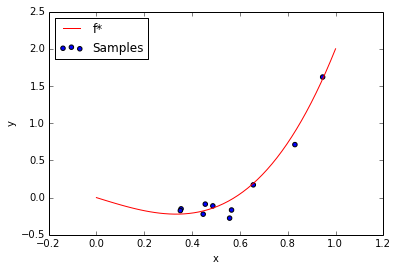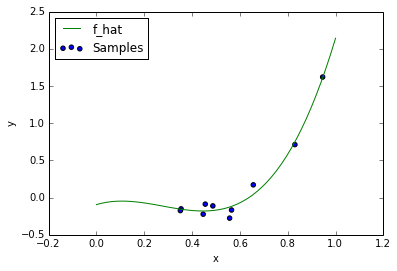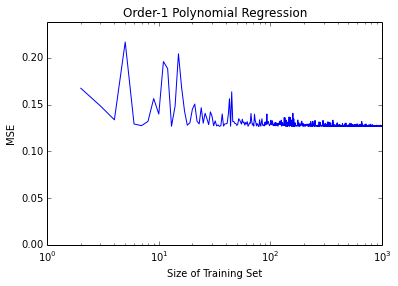CSE 546 Review Session

October 8: Bias-variance trade-off and L1/L2 regularization comparison

In this review section, we'll work through an example to review the bias-variance tradeoff in machine learning. We will later look at some similarities and differences between L1 and L2 regularization.

For this example, assume pairs of data $(x_i, y_i) \in (\mathbb{R}, \mathbb{R})$ are generated from the model $y_i = f^\ast(x_i) + \epsilon_i$. Each $\epsilon_i$ is independently drawn from a Gaussian distribution with mean 0 and standard deviation $\sigma$. For these examples, we will fix $\sigma = 0.1$ and $f^*(x) = 3 x^3 - x$, and we assume each $x_i$ is drawn from a uniform distribution between 0 and 1.

The code below generates a scatter plot of 10 datapoints drawn from this distribution.

In :
import numpy as np
import matplotlib.pyplot as plt

sigma = 0.1

def f_star(x):
return 3 * x**3 - x

def make_data(N):
"""Generates N pairs of data according to y = f*(x) + epsilon"""
x = np.random.rand(N)
noise = sigma * np.random.randn(N)
y = f_star(x) + noise
return (x, y)

# Generate data:
exampleData = make_data(10)

# Plot f_star:
domainSamples = np.linspace(0, 1)
plt.plot(domainSamples, f_star(domainSamples), 'r')

# Plot data:
plt.scatter(exampleData, exampleData)

# Format/show plot:
plt.xlabel('x')
plt.ylabel('y')
plt.legend(('f*', 'Samples'), loc='upper left')
plt.show()Using this model, we will now explore the bias-variance tradeoff using polynomial regression. Given some training data and an order k, we will learn a kth order polynomial function $\hat{f}$ that maps each $x_i$ to a prediction $\hat{y}_i$. The following code illustrates polynomial regression, learning a 4th degree polynomial to fit our 10 example data points.

In :
def expand(x, order):
"""Returns a 2d array with (order + 1) columns.
Column i is equal to x raised to the power i"""
cols = []
for i in range(order + 1):
cols.append(x ** i)
return np.column_stack(cols)

def polynomial_regression((x, y), order):
"""Returns a function learned by polynomial regression of order order"""
lsResult = np.linalg.lstsq(expand(x, order), y)
lsCoefficients = lsResult
return lambda v : np.dot(expand(v, order), lsCoefficients)

f_hat = polynomial_regression(exampleData, order=4)

# Plot f_star:
plt.plot(domainSamples, f_hat(domainSamples), 'g')

# Plot data:
plt.scatter(exampleData, exampleData)

# Format/show plot:
plt.xlabel('x')
plt.ylabel('y')
plt.legend(('f_hat', 'Samples'), loc='upper left')
plt.show()Minimizing Expected Error

Now suppose that our goal is to minimize the expected squared error on some test data. That is, we want to find an $\hat{f}$ that minimizes $R(\hat{f}) = \mathbb{E}_X[(\hat{f}(x) - y)^2] = \mathbb{E}_X[(\hat{f}(x) - f^*(x) - \epsilon)^2]$, where we're taking the expectation over the distribution of $X$.

Since $\epsilon$ is indenpendent from $x$, we can expand $R$ to obtain: $R(\hat{f}) = \mathbb{E}_X[(\hat{f}(x) - f^\ast(x))^2] + \sigma^2$. As a result of our noisy true model, we see that no matter what we choose for $\hat{f}$, we can never expect a loss less than $\sigma^2$.

In machine learning, we learn $\hat{f}$ from data, usually by minimizing the empirical risk on some training data. We assume our training dataset has been created for us by generating random i.i.d. samples $(x_i, y_i)$, and as a result, $\hat{f}$ itself is also random. Thus, the quantity we're actually interested in is $\mathbb{E}_D [\mathbb{E}_X [(\hat{f}(x) - f^*(x))^2]] + \sigma^2$, taking the expected value over a distribution on training data $D$ as well.

In class we saw that the first term can be decomposed into a bias and variance term. Letting $h(x) = \mathbb{E}_D[\hat{f}(x)]$, the function we expect to learn, we end up with

$\mathbb{E}_D [R(\hat{f})] = \mathbb{E}_D \mathbb{E}_X [[(f^*(x) - h(x))^2]] + \mathbb{E}_D \mathbb{E}_X [[(\hat{f}(x) - h(x))^2]] + \sigma^2$

This correspondes to a bias term, a variance term, and a noise term we cannot avoid.

A Biased Hypothesis Class

Let's explore the case when our model of $\hat{f}$ is not complex enough to match the true function $f^\ast$. Specifically, we'll restrict $\hat{f}$ to the form $\hat{f}(x) = mx + b$ by learning only a first degree polynomial. We'll vary the size of the training set, and for each $\hat{f}$, estimate $R(\hat{f})$ empircally with some test data.

In :
# Some test data to empirically evaluate squared error:
testData = make_data(10000)

def get_mse(predictions, testData):
"""Evaluates mean squared error between predictions and test labels"""
yTest = testData
return sum((predictions - yTest) ** 2)/len(yTest)

# Learn a linear model with increasing amounts of training data:
N_ar = range(2, 1000)
mse_ar = []
for N in N_ar:
# Generate N data points:
trainData = make_data(N)

# Perform linear regression:
f_hat = polynomial_regression(trainData, order=1)

# Evaluate:
mse = get_mse(f_hat(testData), testData)
mse_ar.append(mse)

plt.semilogx(N_ar, mse_ar)
plt.ylim(0, 1.1*max(mse_ar))
plt.xlabel('Size of Training Set')
plt.ylabel('MSE')
plt.title('Order-1 Polynomial Regression')
plt.show()

print "Error with", N_ar[-1], "training samples:", mse_ar[-1]Error with 999 training samples: 0.126959275764

Note that even as the size of the training set becomes large, our expected error does not fall below 0.12. This is far from the optimal $\hat{f} = f^\ast$, where the error would approach $\sigma^2 = 0.01$. This result is due to bias. Our model is not complex enough to accurately approximate $f^\ast$.

A More Complex Hypothesis Class

Next we will learn a 4th degree polynomial, but restrict our training set size to only 10 data points. Now the bias is 0, but we are learning 5 coefficients with only 10 datapoints. For comparision, we will also learn a 1st degree polynomial like before. How do you think the results will compare?

In :
# Learn a kth degree polynomial with 10 data points
mse_ar_order1 = []
mse_ar_orderk = []
k = 4 # order of more complex polynomial
N = 10
numTrials = 1000
for trial in range(numTrials):
# Generate N data points:
trainData = make_data(N)

# Perform linear regression:
f_hat_order1 = polynomial_regression(trainData, order=1)
f_hat_orderk = polynomial_regression(trainData, order=k)

# Evaluate:
mse_order1 = get_mse(f_hat_order1(testData), testData)
mse_ar_order1.append(mse_order1)

mse_orderk = get_mse(f_hat_orderk(testData), testData)
mse_ar_orderk.append(mse_orderk)

meanError_order1 = sum(mse_ar_order1)/numTrials
meanError_orderk = sum(mse_ar_orderk)/numTrials

print "Average MSE, 1st degree polynomial:", meanError_order1
print "Average MSE, kth degree polynomial:", meanError_orderk
Average MSE, 1st degree polynomial: 0.173445439906
Average MSE, kth degree polynomial: 1.20624934823

Whoa, the 1st degree polynomial is still better. Why? This is because even though the more complex model now has 0 bias, it large variance. By training on only 10 data points, we still expect the learned model $\hat{f}$ to differ greatly from $f^*$ - even more so than a simpler but biased model. Try increasing the number of training examples N to see what happens then.

L1 vs. L2 Regularization

Least squares with L1 Regularization (Lasso): $\min_{\mathbf{w}, w_0} \sum_i^N (\mathbf{w}^T \mathbf{x_i} + w_0 - y_i)^2 + \lambda ||\mathbf{w}||_1$

Least squares L2 Regularization (Ridge Regression): $\min_{\mathbf{w}, w_0} \sum_i^N (\mathbf{w}^T \mathbf{x_i} + w_0 - y_i)^2 + \lambda||\mathbf{w}||^2_2$

L1, L2, or both:

• Encourages sparsity in solution

• Magnitude of w decreases as lambda increases

• Has a closed form solution

• Larger $\lambda$ helps avoid over-fitting

• Too large a $\lambda$ can lead to under-fitting

• Shrinkage for each weight is the same, regardless of magnitude

• Usually solved with coordinate descent

• Corresponds to MAP estimation with a Gaussian prior

• The same weight can be positive for one value of lambda, zero for a smaller value of lambda

• The same weight can be positive for one value of lambda, negative for a smaller value of lambda

• Tends to lead to better predictive performance

• Used when many more features than examples

• Should generally be debiased after solution is found

• Encourages sparsity in solution - L1

• Magnitude of w decreases as lambda increases - BOTH

• Has a closed form solution - L2

• Larger $\lambda$ helps avoid over-fitting - BOTH

• Too large a $\lambda$ can lead to under-fitting - BOTH

• Shrinkage for each weight is the same, regardless of magnitude - Probably L1, but it depends on the way "shrinkage" is defined. In terms of simply looking at the objective value, it looks like both. In terms of (sub-)gradients and how the shrinkage is applied in an algorithm like coordinate descent, the shrinkage penalty is the same for each parameter, regardless of its current weight. This is the reason L1 encourages sparsity but L2 does not. With L2, we penalize weights more when they're large. With L1, we don't care if they're large or not, the soft-thresholding is the same for everyone, meaning less relevant weights get set exactly 0.

• Usually solved with coordinate descent - L1 (L2 can be solved with coordinate descent too, but a lot of times it's better to use another algorithm)

• Corresponds to MAP estimation with a Gaussian prior - L2

• The same weight can be positive for one value of lambda, zero for a smaller value of lambda - BOTH, but much, much more likely to happen with L1

• The same weight can be positive for one value of lambda, negative for a smaller value of lambda - BOTH

• Tends to lead to better predictive performance - L2 (That is, L2 is often better than L1. This is mainly an empirical observation.)

• Used when many more features than examples - BOTH, but L1 is talked about more often in this case. Really, it depends on what you're trying to do (e.g. if you want sparsity or not).

• Should generally be debiased after solution is found - L1 (L1 squashes the weights a lot to get the desired sparsity - see Murphy page 439).

In []: